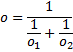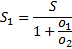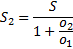betcalcul.com
Betting Calculator

# Double chance bet

Welcome to the world of sports betting mathematics! The first tool of the betting calculator is the double chance bet calculator. It calculates the ratio in which you have to divide your stake between two separate bets in order to have the same return in case of any of the two outcomes. Bookmakers offer double chance odds, which, however, sometimes differ from those calculated from the odds for the two outcomes. With this easy-to-use calculator, you can check that and choose the more advantageous option for you.

Bookmakers usually offer double chance odds for the three combinations — 1X, 12 and X2 — but not always. If the odds for outcome 1 (or 2) are too low (under 1.1, for example), odds for combination 1X (or X2) may not be available because their calculated value is less than 1.00, and betting at odds under 1.00 doesn't make sense.

This calculator is also useful when you want to place two bets on one event at two different bookmakers, taking advantage of the higher odds they are offering for different outcomes and thus obtaining higher effective odds. You could use the calculator when preparing for 1X2 betting, correct score betting, any other type of bet with more than two possible outcomes or a combination of bets of two different types.

Note: Amount calculation performed by the calculator involves rounding. This means that the effective odds for the two outcomes may differ a little. For example, if the calculated double chance odds are 1.5, the effective odds for outcome 1 might be 1.49 and those for the second possible outcome 1.51. The larger the amount you enter, the smaller the rounding error. This rule is valid for all the calculators on this website.

## Formulas for double chance bettingwhere:

• o  - double chance odds
• o1  - odds for outcome 1
• o2  - odds for outcome 2
• S1  - stake for outcome 1
• S2  - stake for outcome 2
• S =S1+S2  - total stake

The odds in the formulas are in decimal format.

## Calculator

Double chance bet calculator
 * Required fields Odds: i Enter the odds (in decimal format) offered for the two outcomes! Stake: i (optional) Enter the total amount that you want to bet on double chance!No connection
18+